### Problem Statement

Onise likes to play with paper and paint. He has a piece of paper with dimensions width x height. He performs K operations, one for each i between 0 and K-1, inclusive. Each operation consists of the following steps:
1. Fold the paper along the line x = xfold[i] (the left side of the paper is folded over the right side).
2. Divide the paper vertically into cnt[i]+1 equal sections. Then, cnt[i] times, take the topmost section and fold it over the section below it.
3. Paint a rectangle with the lower-left corner at (x1[i], y1[i]) and the upper-right corner at (x2[i], y2[i]). Note that (0, 0) is now the lower-left corner of the paper in its current folded state, not its original state. The paint will seep through all the layers of the folded paper. See the image below for clarification.
4. Unfold the paper.
For example, let's say Onise has a piece of paper that is 5 x 6. He performs one operation where xfold is 2, cnt is 2, and the coordinates of the painted rectangle's corners are (1, 1) and (3, 2). The following will happen (note that the paper starts out blue in the images and gets painted white):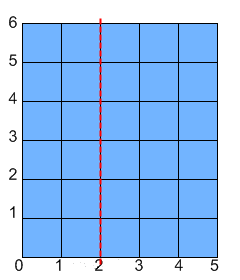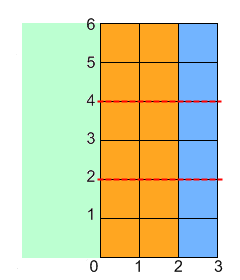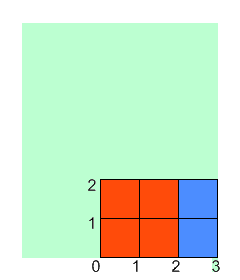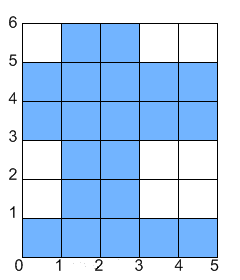You are given ints width and height, and int[]s xfold, cnt, x1, y1, x2 and y2, each containing exactly K elements. Return the total area of of the paper that is not covered in paint after Onise is done.

### Definition

 Class: PaperAndPaint Method: computeArea Parameters: int, int, int[], int[], int[], int[], int[], int[] Returns: long Method signature: long computeArea(int width, int height, int[] xfold, int[] cnt, int[] x1, int[] y1, int[] x2, int[] y2) (be sure your method is public)

### Constraints

-width and height will be between 1 and 10^9, inclusive.
-xfold, cnt, x1, y1, x2 and y2 will all contain the same number of elements, between 1 and 50, inclusive.
-Every element of xfold will be between 0 and width, inclusive.
-Every element of cnt will be between 0 and 1000, inclusive.
-For every i, cnt[i]+1 will be a divisor of height.
-For every i, 0 <= x1[i] < x2[i] <= max(xfold[i], width-xfold[i]) and 0 <= y1[i] < y2[i] <= height/(cnt[i]+1).

### Examples

0)

 `5` `6` `{2}` `{2}` `{1}` `{1}` `{3}` `{2}`
`Returns: 21`
 The example from the problem statement.
1)

 `2` `4` `{0, 0}` `{1, 0}` `{0, 0}` `{1, 1}` `{2, 1}` `{2, 4}`
`Returns: 3`
 Onise will get the following result:2)

 `6` `6` `{2, 5}` `{1, 2}` `{1, 2}` `{2, 0}` `{3, 4}` `{3, 2}`
`Returns: 18`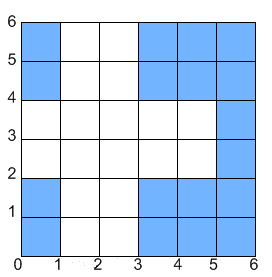3)

 `21` `30` `{3,21,7,11,13}` `{4,14,9,5,4}` `{4,0,2,5,9}` `{2,0,1,2,3}` `{7,19,6,11,12}` `{5,2,2,4,5}`
`Returns: 27`
4)

 `30` `42` `{16, 24, 25, 21, 4}` `{5, 1, 6, 13, 20}` `{3, 1, 5, 8, 9}` `{0, 1, 0, 0, 1}` `{14, 22, 12, 18, 13}` `{2, 15, 1, 1, 2}`
`Returns: 336`
5)

 `26` `60` `{17, 17, 24, 4, 21}` `{4, 1, 11, 0, 2}` `{9, 1, 20, 18, 7}` `{1, 3, 0, 45, 12}` `{13, 4, 23, 19, 13}` `{3, 14, 1, 46, 14}`
`Returns: 1319`
6)

 `17` `3` `{17, 2, 10, 2, 10, 13}` `{2, 0, 0, 2, 0, 0}` `{7, 6, 4, 11, 0, 5}` `{0, 0, 1, 0, 1, 1}` `{12, 10, 6, 12, 4, 12}` `{1, 3, 2, 1, 2, 2}`
`Returns: 20`

#### Problem url:

http://www.topcoder.com/stat?c=problem_statement&pm=10459

#### Problem stats url:

http://www.topcoder.com/tc?module=ProblemDetail&rd=13749&pm=10459

giolekva

#### Testers:

PabloGilberto , lovro , ivan_metelsky

#### Problem categories:

Geometry, Sorting